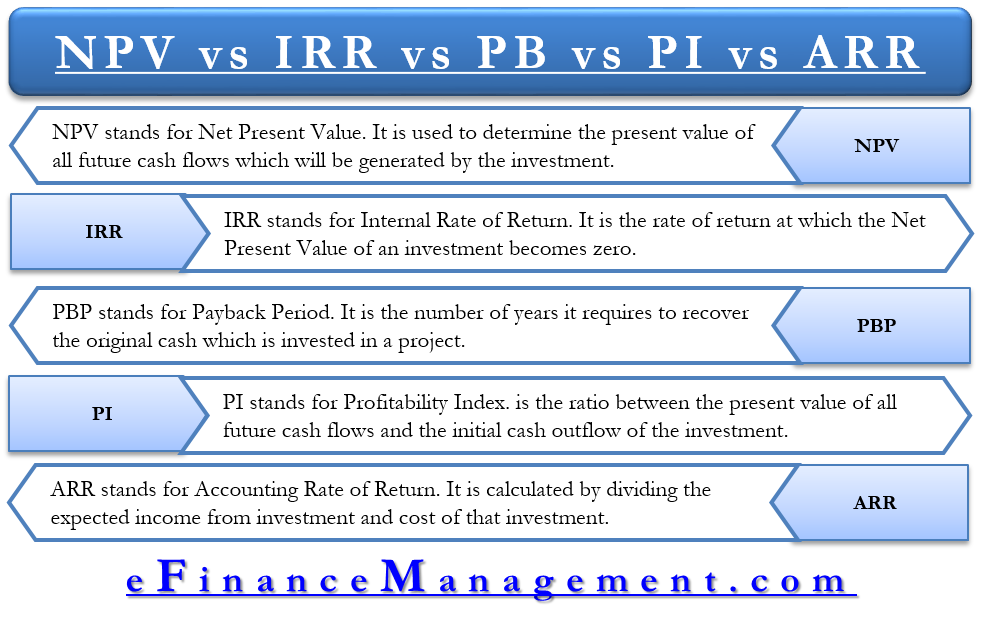# NPV vs IRR vs PB vs PI vs ARR

Every firm has to take several investment decisions in its life. Investment decisions are the firm’s decision to invest its funds, most efficiently, into long term assets. The firms do so because it expects the investment to provide it with some benefits over a single or a series of years. Decisions like launching an advertisement campaign, or a research and development programme has serious implications on the firm because they involve huge money. Hence, a firm must decide its investment only after properly evaluating it.

Several investment criteria, which helps a firm to evaluate investment proposals, are in practice. The most important ones among them are:

Let’s understand each one of them and then we will discuss the difference between them.

## Net Present Value (NPV)

Net present value is a method which is used to determine the present value of all future cash flows which will be generated by the investment. It then compares the present value of all future cash inflows with the value of the cash outflows to decide if the investment should be made or not. Suppose that a project costs you \$1,000 (cash outflow) and it will generate you future cash flows of \$500, \$300 and \$800 in the first, second and third years respectively. Also suppose, that you expect a minimum of 8% return per annum from this investment considering its risk. So, Net Present Value will be the difference between cash outflow and the present value of the future cash inflows. We can calculate it as:

NPV = [\$500/ (1 + 0.08)1 + \$300/(1 + 0.08)2 + \$800/(1 + 0.08)3] – \$1,000

= \$1335.23 – \$1,000

= \$335.23

Considering that the Net Present Value of the investment is positive, the investment proposal should be accepted since this means that the investment is providing more returns than the expected return of 8% per annum. Had the NPV been negative, we would have rejected the proposal since it would have meant that the investment is providing returns lesser than 8% per annum.

## Internal Rate of Return (IRR)

The concept of the Internal Rate of Return is quite simple to understand. Suppose that you invest \$10,000 in a bank today and you will be getting \$10,800 after one year. In this case, IRR will be:

IRR = \$10,800 – \$10,000 / \$10,000

= \$800 / \$10,000

= 8%

IRR, in other words, is the rate of return at which the Net Present Value of an investment becomes zero.

## Payback (PB)

Payback is the number of years it requires to recover the original investment which is invested in a project. If the project generates constant annual cash inflows, we can calculate the payback period as:

Payback = Initial Investment / Annual Cash Inflow## Profitability Index (PI)

Profitability Index is the ratio between the present value of all future cash flows and the initial cash outflow of the investment. If the ratio is greater than 1, then according to the PI method, the company should accept the project since it is providing returns which are greater than the minimum return you expect (used in calculating present value).

PI = Present Value of Future Cash Flows / Initial Cash Outlay

## Accounting Rate of Return (ARR)

Accounting rate of return is also known as the return on investment (ROI). ARR does not consider the time value of money. It is calculated by dividing the income which the company expects to generate from its investment and the cost of that investment.

ARR = (Investment Income / Cost of Investment) * 100

## Differences between the Five Methods

### Point of difference

Net Present Value (NPV)

Internal Rate of Return (IRR)

Payback (PB)

Profitability Index

Accounting Rate of Return

### Meaning

The present value of all future cash flows, less present value of the cash outflowThe rate at which the present value of future cash flows equals the cash outflowThe time within which we will recover the initial cash outflow.The present value of future cash inflow, as the number of times of cash outflowPercentage return on the cash invested.

### Expressed as

We express NPV in the form of currency returns.IRR is expressed in the form of percentage returns.We express PB in the form of a time period.PI is expressed in the form of a ratio.We express ARR in the form of a percentage.

### Focus

NPV focuses on determining whether the investment is generating surplus returns than the expected returns.IRR focuses on determining what is the breakeven rate at which the present value of the future cash flows becomes zero.Payback focuses on determining the time period within which the initial investment can be recovered.PI focuses on determining how many times of the initial investment are we going to get back.Focused on determining the percentage returns from an investment

### Discount Rate

NPV requires the use of a discount rate which can be difficult to ascertain.IRR doesn’t have this difficulty since it ‘calculates’ the rate of return.Payback also does not use discount rates.PI uses a discount rate to discount the future cash flows.ARR does not have the difficulty of ascertaining an appropriate discount rate.

### Calculation of Present Value

NPV calculates the present value of future cash flows.IRR ignores the present value of future cash flows.PB method also ignores the present value of future cash flows.The PI method calculates the present value of future cash flows.ARR does not calculate the present value of future cash flows

123

1.
The difference between NPV and IRR —  AccountingTools. AccountingTools. May 2018. [Source]
2.
Gallant C. Present Value vs Internal Rate of Return. Investopedia. July 2019. [Source]
3.
B Murphy C. What the Accounting Rate of Return Shows. Investopedia. June 2019. [Source]
Last updated on : September 7th, 2019